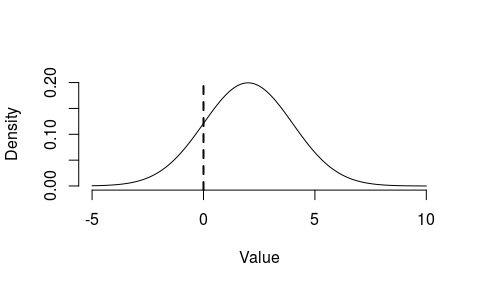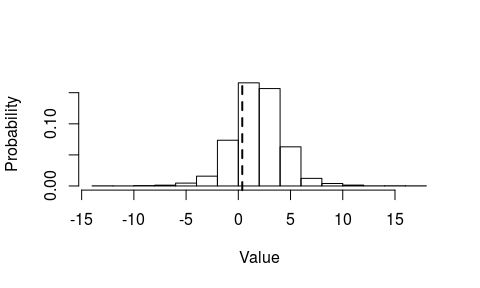# R package scoringRules: Getting started

#### 2018-07-30

This vignette provides a two-page introduction to the scoringRules package. For a more detailed introduction, see the paper ‘Evaluating probabilistic forecasts with scoringRules’ (Jordan, Krüger, and Lerch, Journal of Statistical Software, forthcoming) which is available as a further vignette to scoringRules.

# Background

Forecasting a continuous random variable, such as temperature (in degrees Celsius) or the inflation rate (in percent) is important in many situations. Since forecasts are usually surrounded by uncertainty, it makes sense to state forecast distributions, rather than point forecasts. The scoringRules package offers mathematical tools to evaluate a forecast distribution, $$F$$, after an outcome $$y$$ has realized. To this end, we use scoring rules which assign a penalty score $$S(y, F)$$ to the realization/forecast pair; a smaller score corresponds to a better forecast. See Gneiting and Katzfuss (‘Probabilistic Forecasting’, Annual Review of Statistics and Its Application, 2014) for an introduction to the relevant statistical literature.

scoringRules supports two types of forecast distributions, $$F$$: Distributions given by a parametric family (such as Normal or Gamma), and distributions given by a simulated sample. Furthermore, the package covers the two most popular scoring rules $$S$$: The logarithmic score (LogS), given by $\text{LogS}(y, F) = -\log f(y),$ where $$f$$ is the density conforming to $$F$$, and the continuous ranked probability score (CRPS), given by $\text{CRPS}(y, F) = \int_{-\infty}^\infty (F(z) - \mathbf{1}(y \le z))^2 dz,$ where $$\mathbf{1}(A)$$ is the indicator function of the event $$A$$. The LogS is typically easy to compute, and we include it mainly for reference purposes. By contrast, the CRPS can be very tricky to compute analytically, and cumbersome to approximate numerically. The scoringRules package implements several new methods to tackle this challenge. Specifically, we include many previously unknown analytical expressions, and incorporate recent findings on how to best compute the CRPS of a simulated forecast distribution.

# Example 1: Parametric forecast distribution

Suppose the forecast distribution is $$\mathcal{N}(2, 4)$$, and an outcome of zero realizes:The following piece of code computes the CRPS for this situation:

library(scoringRules)
# CRPS of a normal distribution with mean = standard deviation = 2, outcome is zero
crps(y = 0, family = "normal", mean = 2, sd = 2)
##  1.204883

As documented under ?crps, the parametric family used in the example is one of the following choices currently implemented in scoringRules:

• Normal, Mixture of normals, Two-piece normal, Log-normal, Generalized truncated/censored normal, (Scaled) Student t, Generalized truncated/censored Student t, Laplace, Log-Laplace, Logistic, Log-logistic, Generalized truncated/censored logistic, Exponential, Exponential with point mass, Two-piece exponential, Beta, Gamma, Uniform, Generalized extreme value, Generalized Pareto, Binomial, Hypergeometric, Negative binomial, Poisson

We provide analytical CRPS formulas in Appendix A of the vignette ‘Evaluating Probabilistic Forecasts with scoringRules’. Whenever possible, our syntax and parametrization closely follow base R. For distributions not supported by base R, we have created documentation pages with details; see for example ?f2pnorm.

# Example 2: Simulated forecast distribution

Via data(gdp_mcmc), the user can load a sample data set with forecasts and realizations for the growth rate of US gross domestic product, a widely regarded economic indicator. The following plot shows the histogram of a simulated forecast distribution for the fourth quarter of 2012:Let’s now compute the CRPS for this simulated forecast distribution:

# Load data
data(gdp_mcmc)
# Get forecast distribution for 2012:Q4
dat <- gdp_mcmc$forecasts[, "X2012Q4"] # Get realization for 2012:Q4 y <- gdp_mcmc$actuals[, "X2012Q4"]
# Compute CRPS of simulated sample
crps_sample(y = y, dat = dat)
##  0.9058803